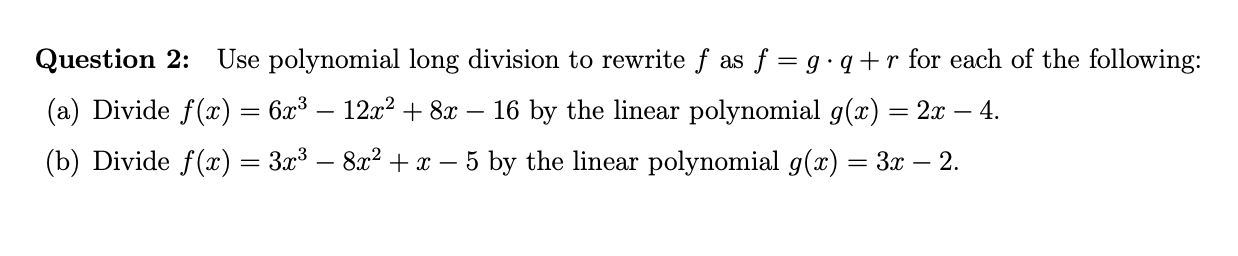# Use polynomial long division to rewrite f as f = g q+ r for each of the followingQuestion 2:(a) Divide f(x) = 6x3 - 12x2 +8x- 16 by the linear polynomial g(x) = 2x - 4.(b) Divide f(z) = 3x3 - 8x2+x - 5 by the linear polynomial g(x)= 3x - 2.

Question
6 viewshelp_outlineImage TranscriptioncloseUse polynomial long division to rewrite f as f = g q+ r for each of the following Question 2: (a) Divide f(x) = 6x3 - 12x2 +8x - 16 by the linear polynomial g(x) = 2x - 4. (b) Divide f(z) = 3x3 - 8x2+x - 5 by the linear polynomial g(x)= 3x - 2. fullscreen
check_circle

Step 1

According to the given question in part (a):

Step 2

Now divide f(x) by g(x) using long division:

Step 3

Now,

R(x) = 0

Q(x) = 3x2+4

G(x) = 2x-4

So, f(x) = (2x-...

### Want to see the full answer?

See Solution

#### Want to see this answer and more?

Solutions are written by subject experts who are available 24/7. Questions are typically answered within 1 hour.*

See Solution
*Response times may vary by subject and question.
Tagged in

### Other